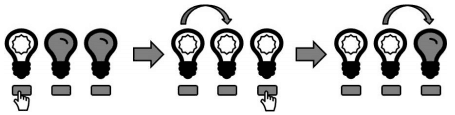### C - Flashing Fluorescents

##### Languages: C, C++, Java, Python, ... (details)

You have $n$ lights, each with its own button, in a line. Pressing a light’s button will toggle that light’s state; if the light is on, it will turn off, and if the light is off, it will turn on. The lights change at $1$ second timesteps. You can press a button at any time, but it will not take effect until the next timestep. Before each timestep, you may choose to push at most one button (you may also choose to not press any button).

Pushing a button will affect not just the light in question, but all lights down the line. More specifically, if you choose to press the $i^{th}$ button right before the $k^{th}$ timestep, then the $(i + m)^{th}$ light will toggle on the $(k + m)^{th}$ timestep (with $i + m \leq n$). For example, if you press button $5$ just before time $19$, then light $5$ will toggle at time $19$, light $6$ will toggle at time $20$, light $7$ will toggle at time $21$, and so on. If you push a button that will take effect at the same time as its light would have toggled due to an earlier button press, then the two cancel each other out, including subsequent toggles.

Suppose there are three lights, all of which are off at the start. If you press the first button before the first timestep, this will happen in three timesteps:Now, suppose you press the first button before the first timestep, and then the second button between the first and second timesteps. The button press will cancel out the propagation, and this will happen (note that the propagation will go no further):Now, suppose you press the first button before the first timestep, and then the third button between the first and second timesteps. All three lights will be on at the second timestep (but not the third):You wish to turn on all the lights. What is the earliest time you could possibly see all of the lights turned on? Note that if the lights are all on at time t but not at time $t + 1$ due to this propagation, $t$ is still the correct answer

#### Input

Each input will consist of a single test case. Note that your program may be run multiple times on different inputs. Each test case will consist of a single string $S$ $(1 \leq |S| \leq 16)$. The string $S$ will contain only the characters $1$ and $0$, where $1$ represents that that light is initially on, and $0$ represents that that light is initially off. The first character is light $1$, the next is light $2$, and so on.

#### Output

Output a single integer, which is the earliest time at which all of the lights are on.

Input
1101
Output
1
Input
1
Output
0
Input
000
Output
2